Скачать презентацию Oligopoly and Strategic Interaction 1 Introduction

05b52fd990f6e7ca1d9aed3514376e46.ppt

• Количество слайдов: 63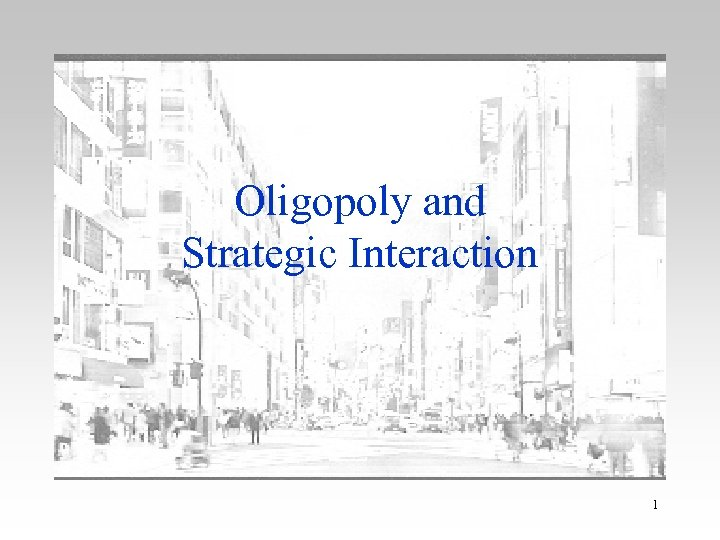Oligopoly and Strategic Interaction 1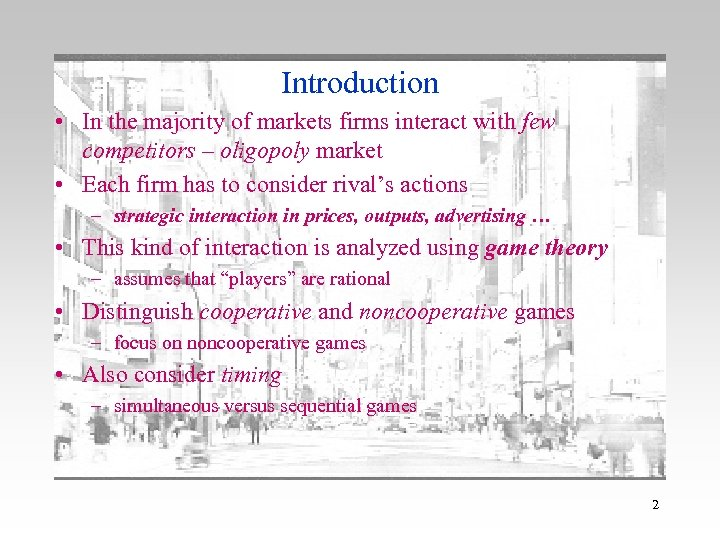Introduction • In the majority of markets firms interact with few competitors – oligopoly market • Each firm has to consider rival’s actions – strategic interaction in prices, outputs, advertising … • This kind of interaction is analyzed using game theory – assumes that “players” are rational • Distinguish cooperative and noncooperative games – focus on noncooperative games • Also consider timing – simultaneous versus sequential games 2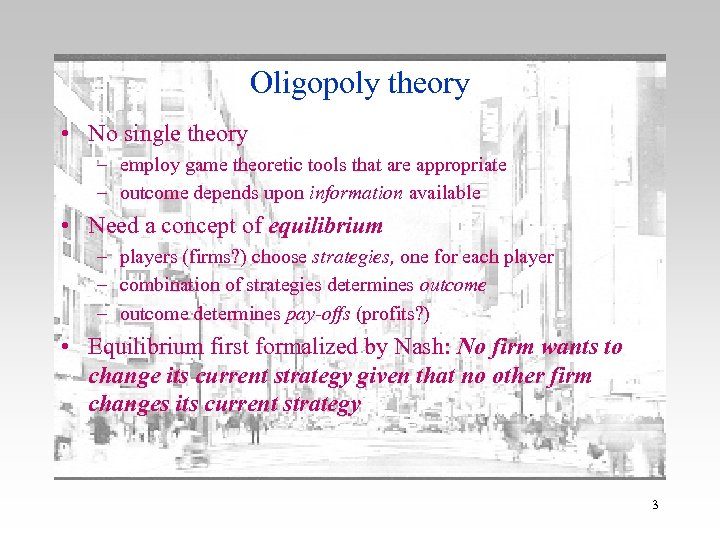Oligopoly theory • No single theory – employ game theoretic tools that are appropriate – outcome depends upon information available • Need a concept of equilibrium – players (firms? ) choose strategies, one for each player – combination of strategies determines outcome – outcome determines pay-offs (profits? ) • Equilibrium first formalized by Nash: No firm wants to change its current strategy given that no other firm changes its current strategy 3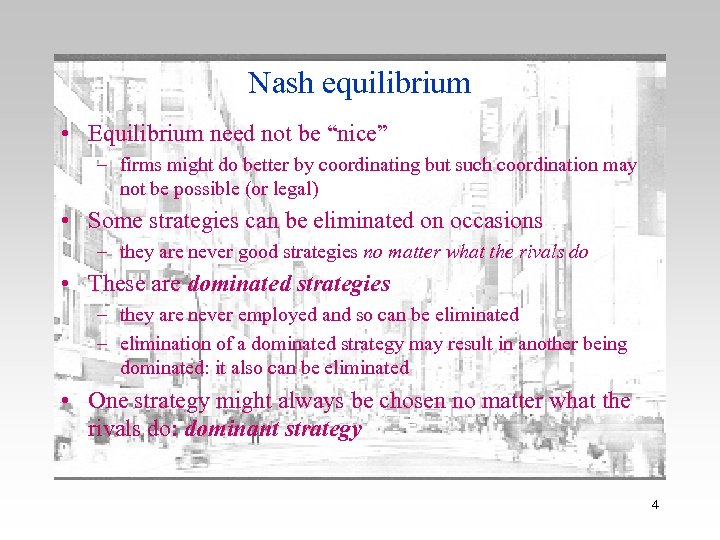Nash equilibrium • Equilibrium need not be “nice” – firms might do better by coordinating but such coordination may not be possible (or legal) • Some strategies can be eliminated on occasions – they are never good strategies no matter what the rivals do • These are dominated strategies – they are never employed and so can be eliminated – elimination of a dominated strategy may result in another being dominated: it also can be eliminated • One strategy might always be chosen no matter what the rivals do: dominant strategy 4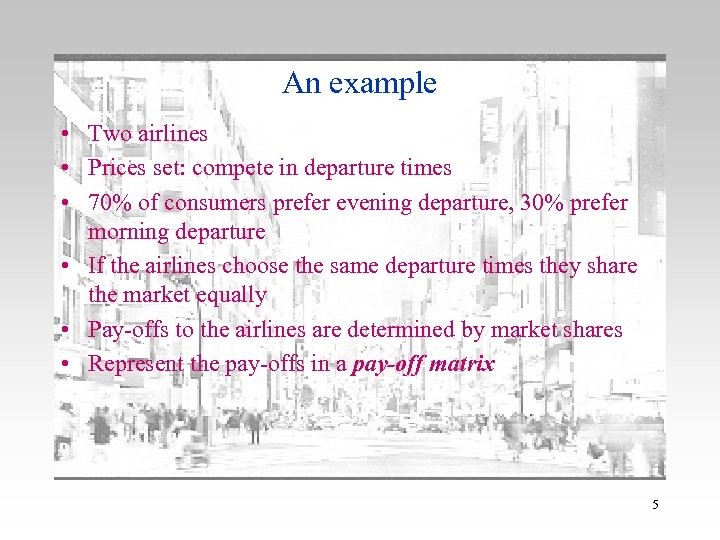An example • Two airlines • Prices set: compete in departure times • 70% of consumers prefer evening departure, 30% prefer morning departure • If the airlines choose the same departure times they share the market equally • Pay-offs to the airlines are determined by market shares • Represent the pay-offs in a pay-off matrix 5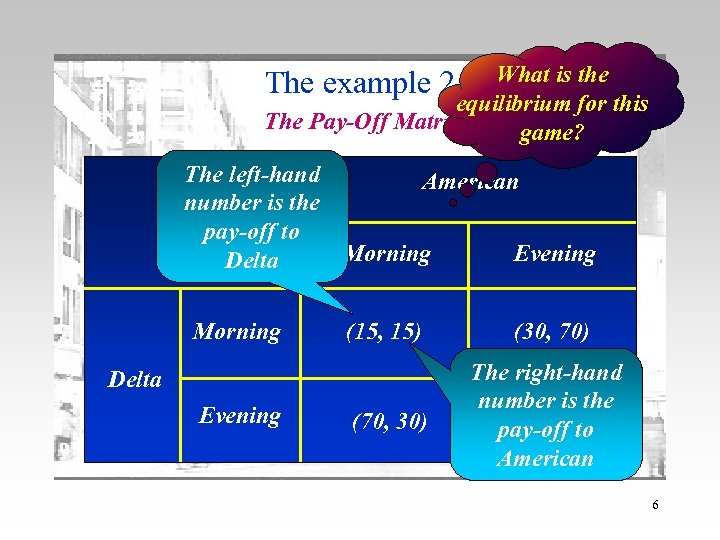What is the equilibrium for this The Pay-Off Matrix game? The example 2 The left-hand American number is the pay-off to Morning Evening Delta Morning (15, 15) (30, 70) (70, 30) The right-hand number is the (35, 35) pay-off to American Delta Evening 6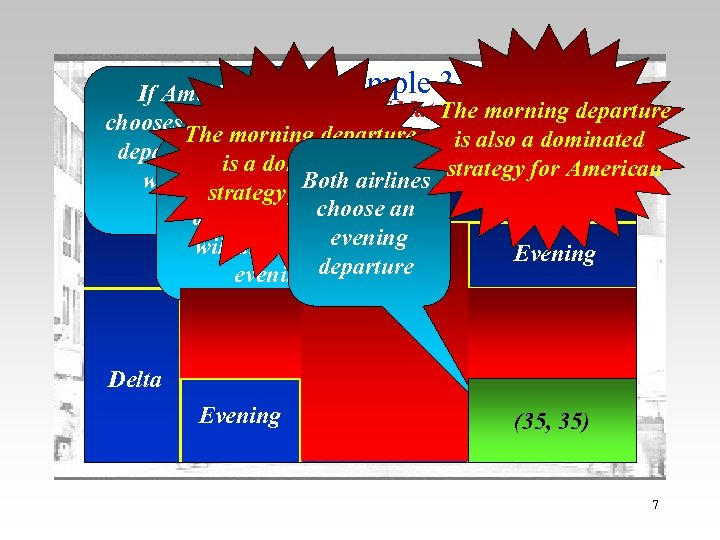If American The example 3 The Pay-Off Matrix morning departure The chooses a morning The morning departure is also a dominated departure, Delta If American is a dominated Both airlines strategy for American willchooses an evening choose American strategy for Delta choose an evening departure, Delta evening will also choose Morning Evening departure evening Morning (15, 15) (30, 70) Evening (70, 30) (35, 35) Delta 7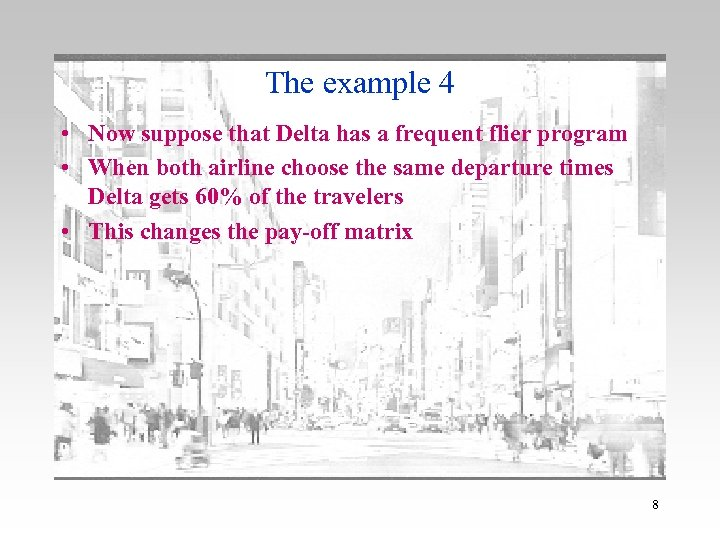The example 4 • Now suppose that Delta has a frequent flier program • When both airline choose the same departure times Delta gets 60% of the travelers • This changes the pay-off matrix 8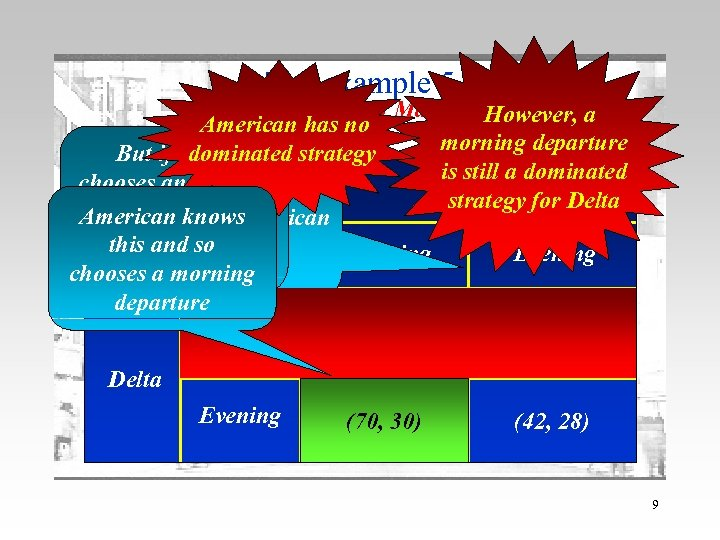The example 5 The Pay-Off Matrix However, a American has no morning departure dominated strategy But if Delta If Delta is still a American dominated chooses an evening chooses a morning strategy for Delta departure, American knows departure, American will choose this and sochoose will Morning Evening morning chooses a morning evening departure Morning (18, 12) (30, 70) Delta Evening (70, 30) (42, 28) 9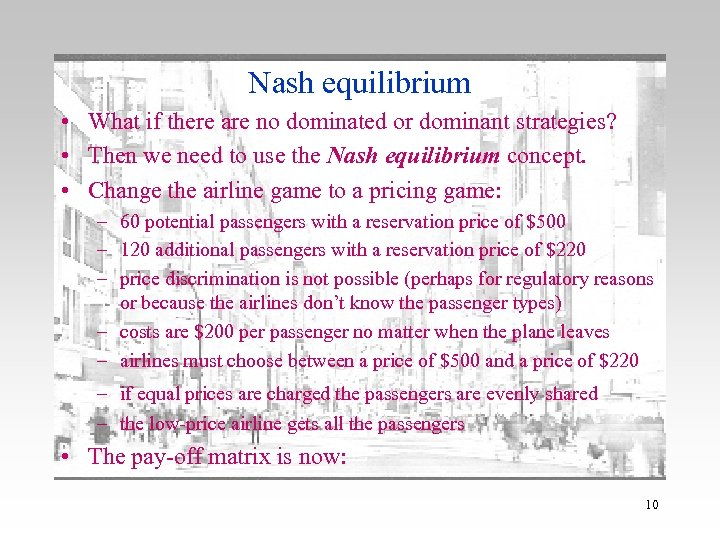Nash equilibrium • What if there are no dominated or dominant strategies? • Then we need to use the Nash equilibrium concept. • Change the airline game to a pricing game: – 60 potential passengers with a reservation price of \$500 – 120 additional passengers with a reservation price of \$220 – price discrimination is not possible (perhaps for regulatory reasons or because the airlines don’t know the passenger types) – costs are \$200 per passenger no matter when the plane leaves – airlines must choose between a price of \$500 and a price of \$220 – if equal prices are charged the passengers are evenly shared – the low-price airline gets all the passengers • The pay-off matrix is now: 10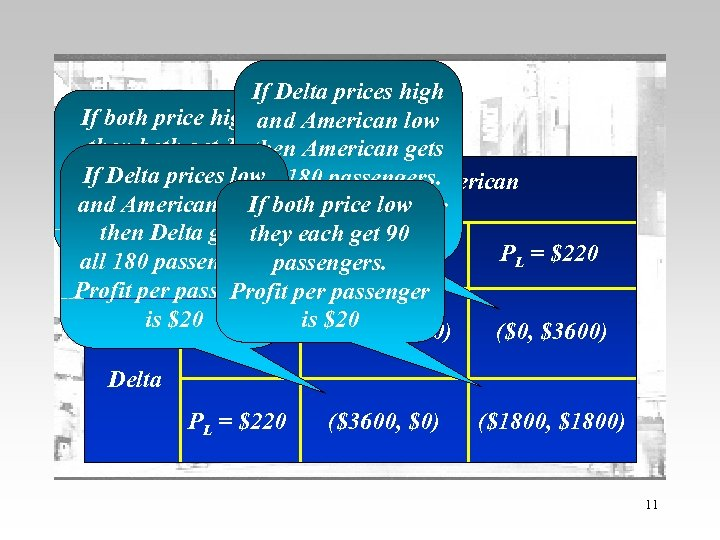The example If Delta prices high The Pay-Off low If both price high and American. Matrix then both get 30 then American gets passengers. Profitall 180 passengers. If Delta prices low American per passenger is Profit price low and American high bothper passenger If then\$300 gets they each\$20 90 Delta is get PH = PL = \$220 all 180 passengers. \$500 Profit per passenger Profit is \$20 = \$500 is \$20 P (\$9000, \$9000) (\$0, \$3600) H Delta PL = \$220 (\$3600, \$0) (\$1800, \$1800) 11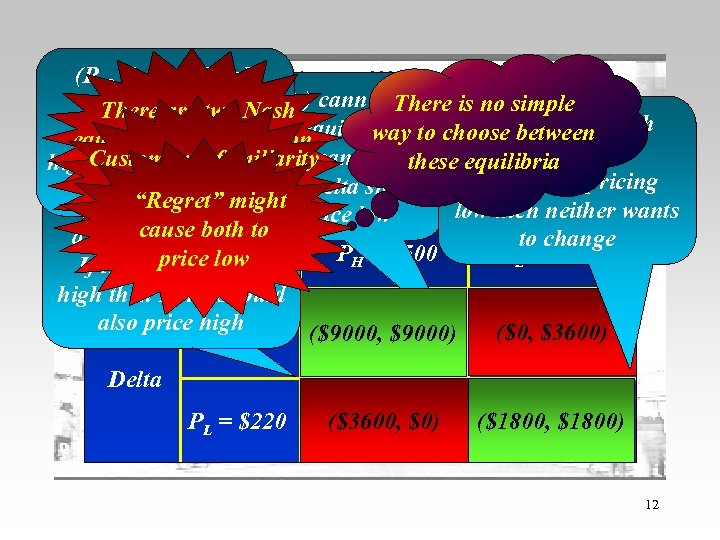(PH, PH) is a Nash cannot equilibrium (PHThe )Pay-Off be , PL There is no simple equilibrium. Matrix There are two Nash (PL PL) is a a version Nash equilibrium. choose , between Nash way to If both are pricing equilibria to this equilibrium. If American prices these equilibria high. Custom and familiarity then neither wants of the game If both American are pricing might lead low then Delta should to“Regret”both to change might (PL, PHprice high also price low ) cannot be low then neither wants a Nashcause both to equilibrium. to change PH = \$500 PL = \$220 price low If American prices high then Delta should also price high PH = \$500 (\$9000, \$9000) (\$0, \$3600) Delta PL = \$220 (\$3600, \$0) (\$1800, \$1800) 12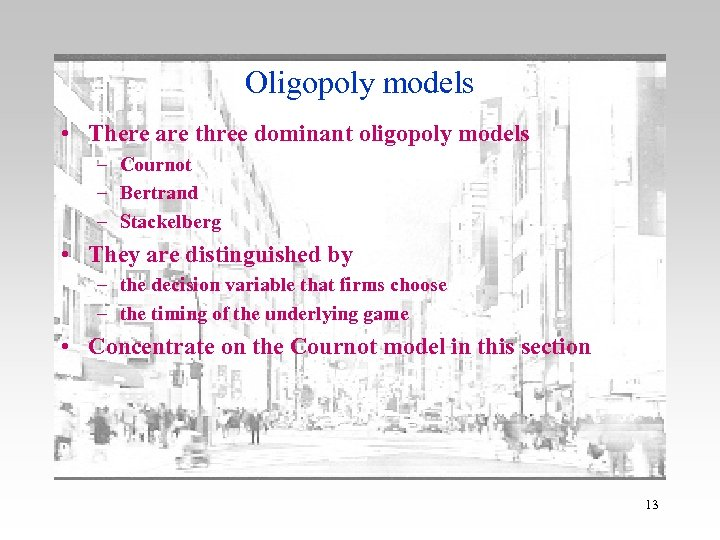Oligopoly models • There are three dominant oligopoly models – Cournot – Bertrand – Stackelberg • They are distinguished by – the decision variable that firms choose – the timing of the underlying game • Concentrate on the Cournot model in this section 13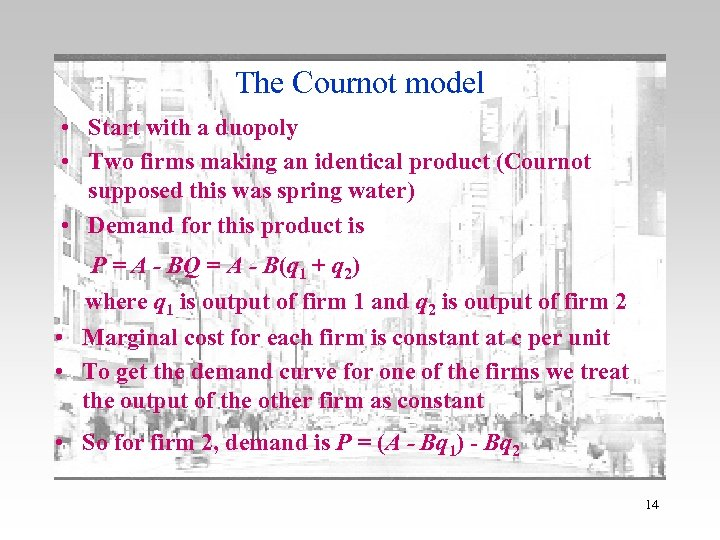The Cournot model • Start with a duopoly • Two firms making an identical product (Cournot supposed this was spring water) • Demand for this product is P = A - BQ = A - B(q 1 + q 2) where q 1 is output of firm 1 and q 2 is output of firm 2 • Marginal cost for each firm is constant at c per unit • To get the demand curve for one of the firms we treat the output of the other firm as constant • So for firm 2, demand is P = (A - Bq 1) - Bq 2 14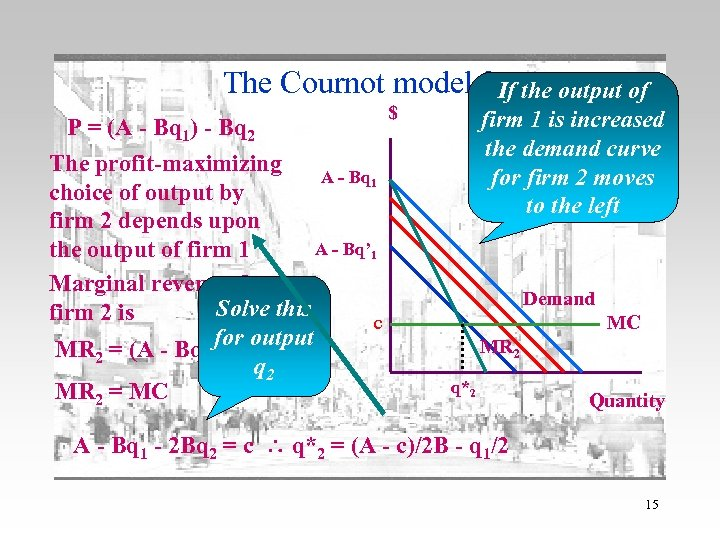The Cournot model 2 If the output of P = (A - Bq 1) - Bq 2 The profit-maximizing A - Bq 1 choice of output by firm 2 depends upon A - Bq’ 1 the output of firm 1 Marginal revenue for Solve this firm 2 is c for output MR 2 = (A - Bq 1) - 2 Bq 2 MR 2 = MC \$ firm 1 is increased the demand curve for firm 2 moves to the left Demand MC MR 2 q*2 Quantity A - Bq 1 - 2 Bq 2 = c q*2 = (A - c)/2 B - q 1/2 15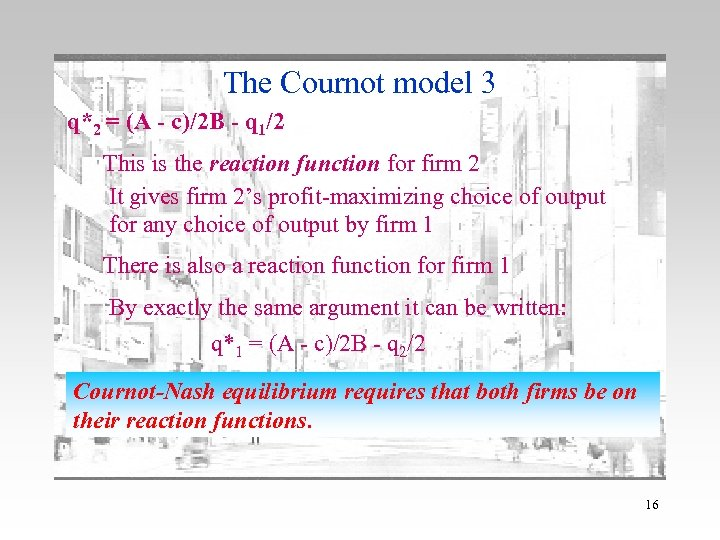The Cournot model 3 q*2 = (A - c)/2 B - q 1/2 This is the reaction function for firm 2 It gives firm 2’s profit-maximizing choice of output for any choice of output by firm 1 There is also a reaction function for firm 1 By exactly the same argument it can be written: q*1 = (A - c)/2 B - q 2/2 Cournot-Nash equilibrium requires that both firms be on their reaction functions. 16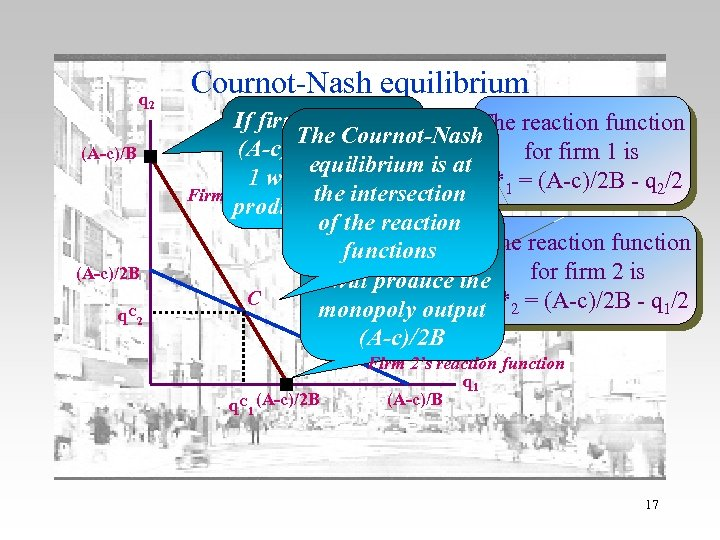q 2 (A-c)/B (A-c)/2 B q. C 2 Cournot-Nash equilibrium If firm 2 produces The reaction function The Cournot-Nash (A-c)/B then firm for firm 1 is equilibrium is at 1 will choose to q*1 = (A-c)/2 B - q 2/2 Firm 1’s reactionthe intersection function produce no output of the 2 produces If firm reaction The reaction functions nothing then firm for firm 2 is 1 will produce the C monopoly output q*2 = (A-c)/2 B - q 1/2 (A-c)/2 B q. C 1 (A-c)/2 B Firm 2’s reaction function q 1 (A-c)/B 17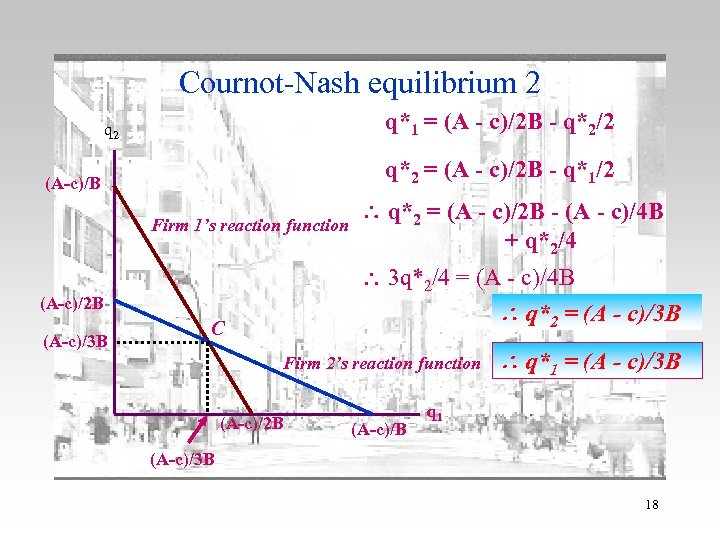Cournot-Nash equilibrium 2 q*1 = (A - c)/2 B - q*2/2 q 2 q*2 = (A - c)/2 B - q*1/2 (A-c)/B Firm 1’s reaction function 3 q*2/4 = (A - c)/4 B q*2 = (A - c)/3 B (A-c)/2 B (A-c)/3 B q*2 = (A - c)/2 B - (A - c)/4 B + q*2/4 C Firm 2’s reaction function (A-c)/2 B (A-c)/B q*1 = (A - c)/3 B q 1 (A-c)/3 B 18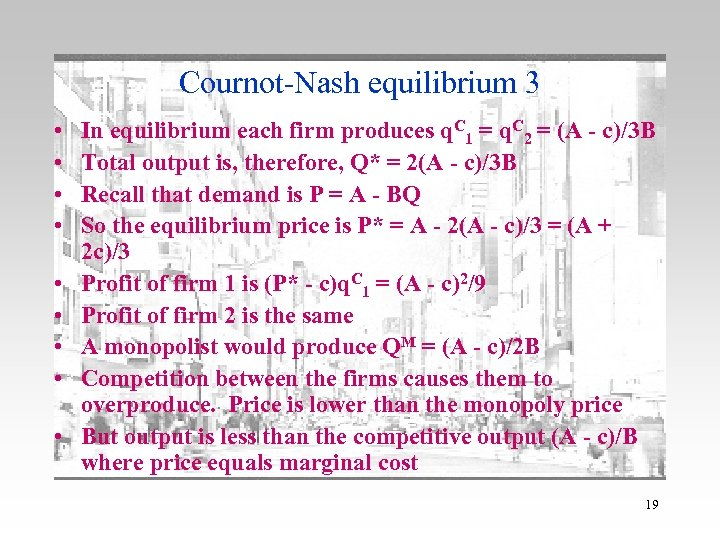Cournot-Nash equilibrium 3 • • • In equilibrium each firm produces q. C 1 = q. C 2 = (A - c)/3 B Total output is, therefore, Q* = 2(A - c)/3 B Recall that demand is P = A - BQ So the equilibrium price is P* = A - 2(A - c)/3 = (A + 2 c)/3 Profit of firm 1 is (P* - c)q. C 1 = (A - c)2/9 Profit of firm 2 is the same A monopolist would produce QM = (A - c)/2 B Competition between the firms causes them to overproduce. Price is lower than the monopoly price But output is less than the competitive output (A - c)/B where price equals marginal cost 19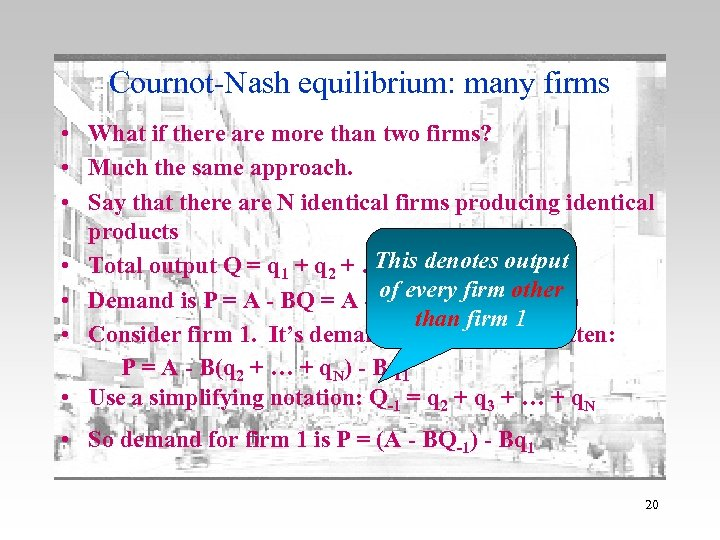Cournot-Nash equilibrium: many firms • What if there are more than two firms? • Much the same approach. • Say that there are N identical firms producing identical products This N • Total output Q = q 1 + q 2 + … + qdenotes output of every firm other • Demand is P = A - BQ = A - B(q 1 + q 2 + … + q. N) than firm 1 • Consider firm 1. It’s demand curve can be written: P = A - B(q 2 + … + q. N) - Bq 1 • Use a simplifying notation: Q-1 = q 2 + q 3 + … + q. N • So demand for firm 1 is P = (A - BQ-1) - Bq 1 20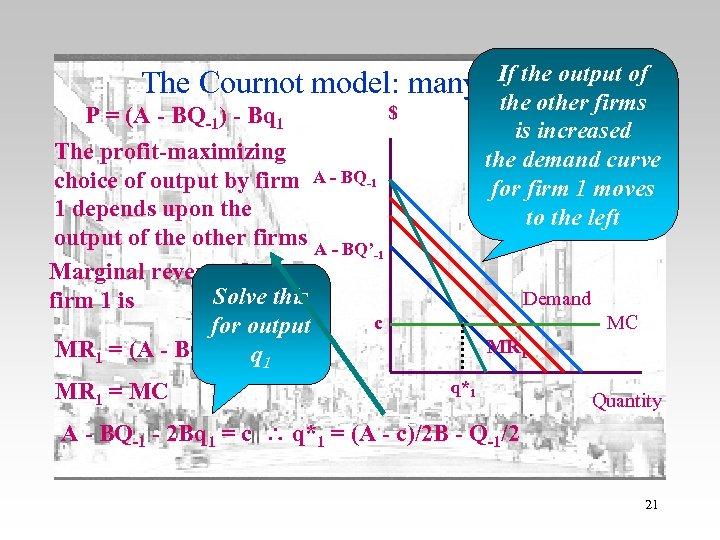If the 2 The Cournot model: many firms output of P = (A - BQ-1) - Bq 1 the other firms is increased the demand curve for firm 1 moves to the left \$ The profit-maximizing choice of output by firm A - BQ-1 1 depends upon the output of the other firms A - BQ’ -1 Marginal revenue for Solve this firm 1 is c for output MR 1 = (A - BQ-1) - 2 Bq 1 MR 1 = MC Demand MC MR 1 q*1 Quantity A - BQ-1 - 2 Bq 1 = c q*1 = (A - c)/2 B - Q-1/2 21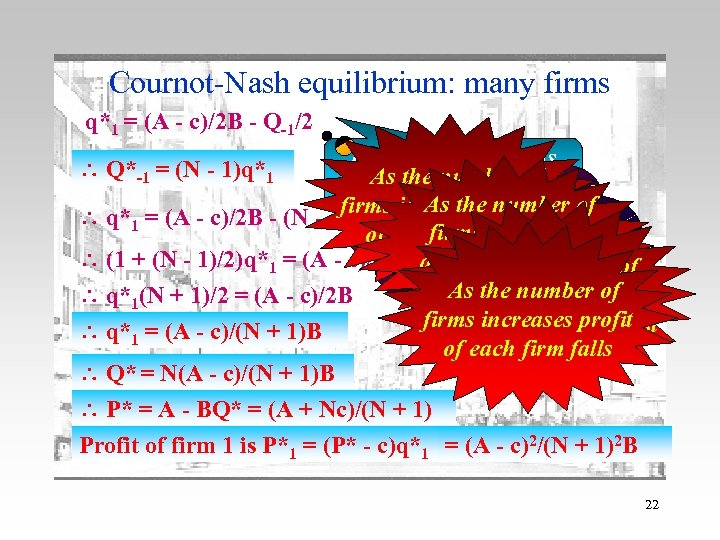Cournot-Nash equilibrium: many firms q*1 = (A - c)/2 B - Q-1/2 How do we solve this As the number of for q*1? The firms are identical. As the output firms increases number of q*1 = (A - c)/2 B - (N - 1)q*1/2 in equilibrium they So firms of each firm increases falls will have identical (1 + (N - 1)/2)q*1 = (A - c)/2 B aggregate output of As the number outputs increases As the number of firms increases price q*1(N + 1)/2 = (A - c)/2 B firms increases profit tends to marginal cost q*1 = (A - c)/(N + 1)B of each firm falls Q* = N(A - c)/(N + 1)B P* = A - BQ* = (A + Nc)/(N + 1) Profit of firm 1 is P*1 = (P* - c)q*1 = (A - c)2/(N + 1)2 B Q*-1 = (N - 1)q*1 22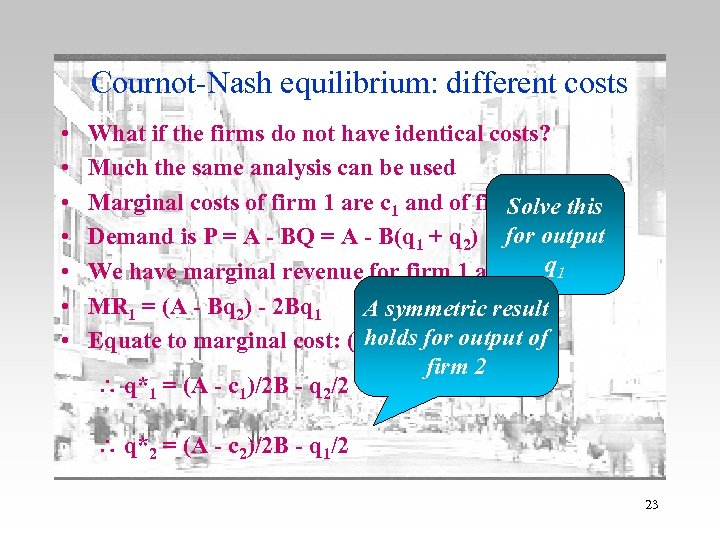Cournot-Nash equilibrium: different costs • • What if the firms do not have identical costs? Much the same analysis can be used Marginal costs of firm 1 are c 1 and of firm 2 are c 2. Solve this Demand is P = A - BQ = A - B(q 1 + q 2) for output q 1 We have marginal revenue for firm 1 as before MR 1 = (A - Bq 2) - 2 Bq 1 A symmetric result holds for output c 1 Equate to marginal cost: (A - Bq 2) - 2 Bq 1 = of firm 2 q*1 = (A - c 1)/2 B - q 2/2 q*2 = (A - c 2)/2 B - q 1/2 23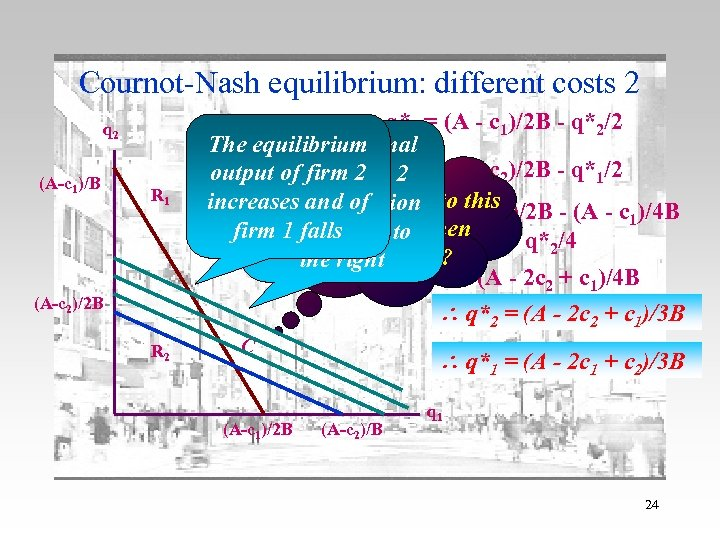Cournot-Nash equilibrium: different costs 2 q 2 (A-c 1)/B R 1 (A-c 2)/2 B R 2 q*1 = (A - c 1)/2 B - q*2/2 The equilibrium If the marginal output cost of firmq*2 = (A - c 2)/2 B - q*1/2 of firm 2 2 What happens increases andreaction =to this )/2 B - (A - c )/4 B falls its of q* (A - c 2 2 1 firmcurve shifts to when + q* /4 1 equilibrium falls 2 costs change? the right 3 q*2/4 = (A - 2 c 2 + c 1)/4 B q*2 = (A - 2 c 2 + c 1)/3 B C (A-c 1)/2 B q*1 = (A - 2 c 1 + c 2)/3 B (A-c 2)/B q 1 24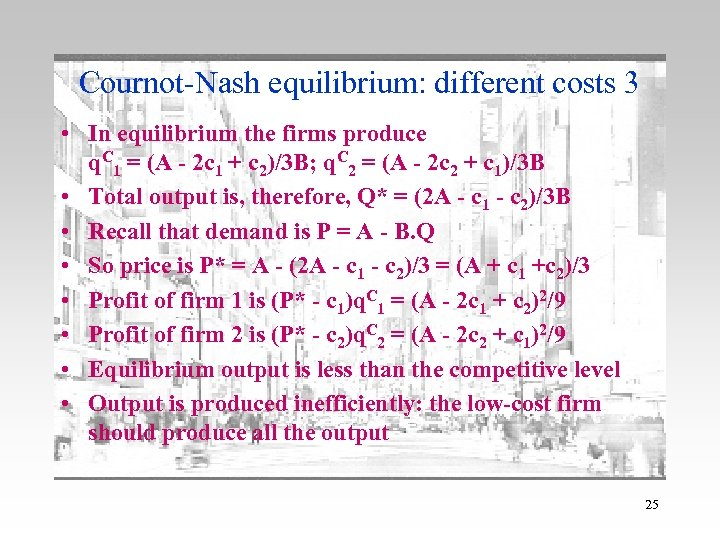Cournot-Nash equilibrium: different costs 3 • In equilibrium the firms produce q. C 1 = (A - 2 c 1 + c 2)/3 B; q. C 2 = (A - 2 c 2 + c 1)/3 B • Total output is, therefore, Q* = (2 A - c 1 - c 2)/3 B • Recall that demand is P = A - B. Q • So price is P* = A - (2 A - c 1 - c 2)/3 = (A + c 1 +c 2)/3 • Profit of firm 1 is (P* - c 1)q. C 1 = (A - 2 c 1 + c 2)2/9 • Profit of firm 2 is (P* - c 2)q. C 2 = (A - 2 c 2 + c 1)2/9 • Equilibrium output is less than the competitive level • Output is produced inefficiently: the low-cost firm should produce all the output 25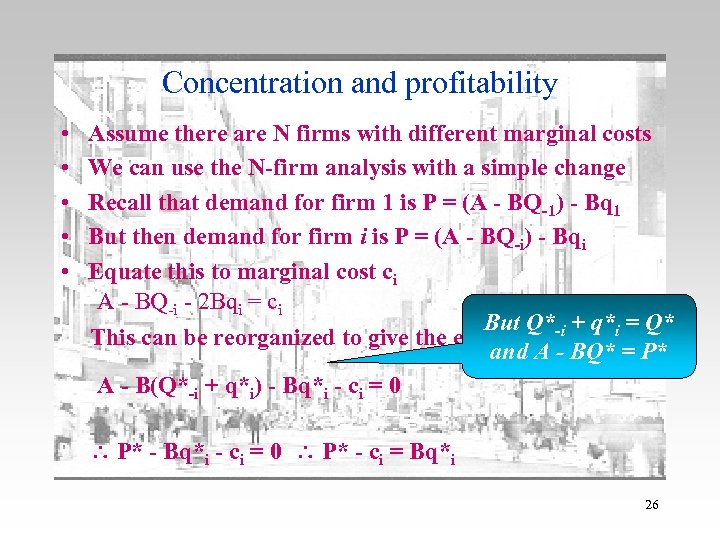Concentration and profitability • • • Assume there are N firms with different marginal costs We can use the N-firm analysis with a simple change Recall that demand for firm 1 is P = (A - BQ-1) - Bq 1 But then demand for firm i is P = (A - BQ-i) - Bqi Equate this to marginal cost ci A - BQ-i - 2 Bqi = ci But Q*-i + q*i = Q* This can be reorganized to give the equilibrium condition: and A - BQ* = P* A - B(Q*-i + q*i) - Bq*i - ci = 0 P* - ci = Bq*i 26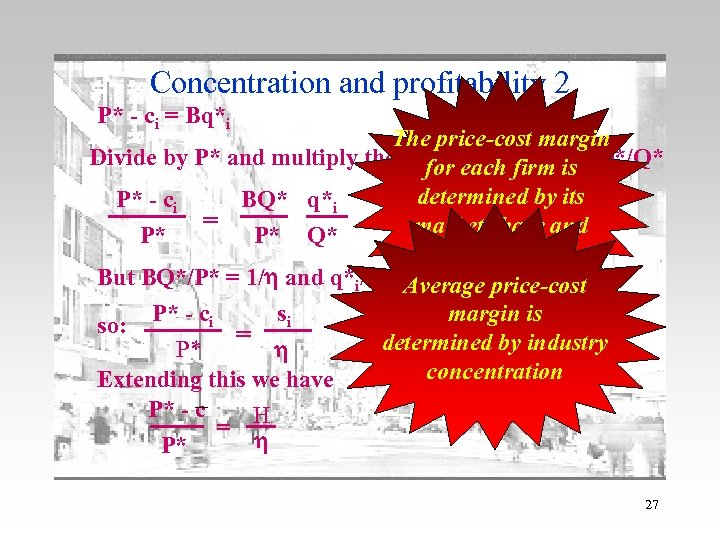Concentration and profitability 2 P* - ci = Bq*i The price-cost margin Divide by P* and multiply the right-handfirm is Q*/Q* for each side by determined by its P* - ci BQ* q*i = market share and P* P* Q* demand elasticity But BQ*/P* = 1/ and q*i/Q* = si Average price-cost margin is so: P* - ci = si determined by industry P* concentration Extending this we have P* - c = H P* 27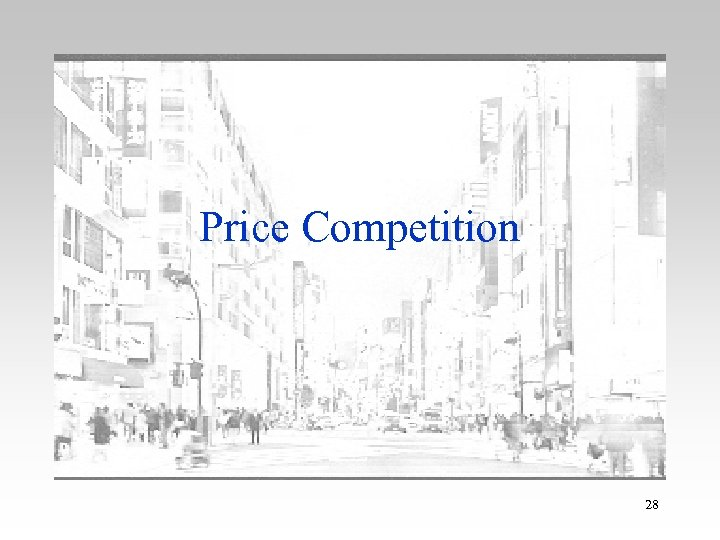Price Competition 28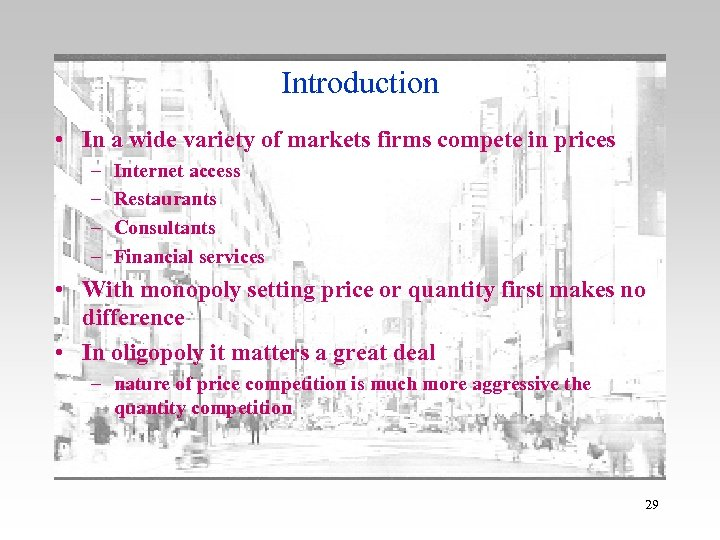Introduction • In a wide variety of markets firms compete in prices – – Internet access Restaurants Consultants Financial services • With monopoly setting price or quantity first makes no difference • In oligopoly it matters a great deal – nature of price competition is much more aggressive the quantity competition 29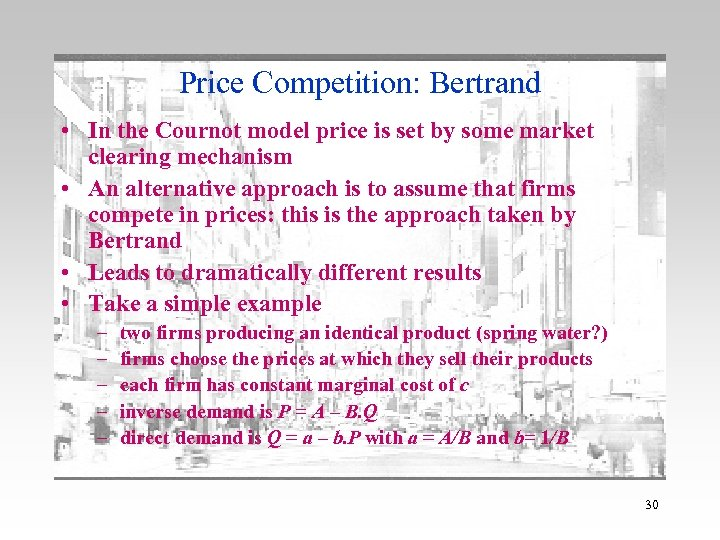Price Competition: Bertrand • In the Cournot model price is set by some market clearing mechanism • An alternative approach is to assume that firms compete in prices: this is the approach taken by Bertrand • Leads to dramatically different results • Take a simple example – – – two firms producing an identical product (spring water? ) firms choose the prices at which they sell their products each firm has constant marginal cost of c inverse demand is P = A – B. Q direct demand is Q = a – b. P with a = A/B and b= 1/B 30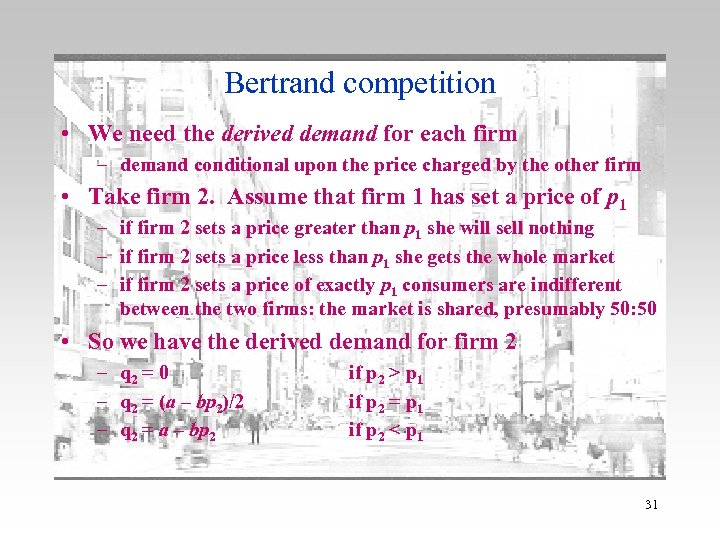Bertrand competition • We need the derived demand for each firm – demand conditional upon the price charged by the other firm • Take firm 2. Assume that firm 1 has set a price of p 1 – if firm 2 sets a price greater than p 1 she will sell nothing – if firm 2 sets a price less than p 1 she gets the whole market – if firm 2 sets a price of exactly p 1 consumers are indifferent between the two firms: the market is shared, presumably 50: 50 • So we have the derived demand for firm 2 – q 2 = 0 – q 2 = (a – bp 2)/2 – q 2 = a – bp 2 if p 2 > p 1 if p 2 = p 1 if p 2 < p 1 31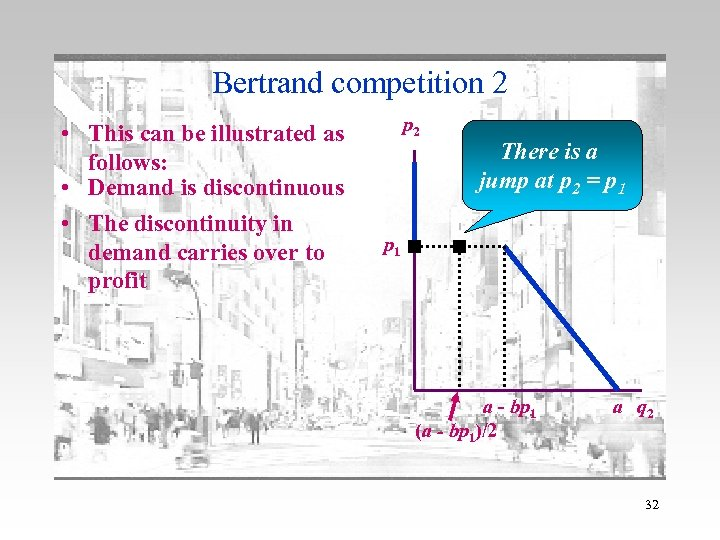Bertrand competition 2 • This can be illustrated as follows: • Demand is discontinuous • The discontinuity in demand carries over to profit p 2 There is a jump at p 2 = p 1 a - bp 1 (a - bp 1)/2 a q 2 32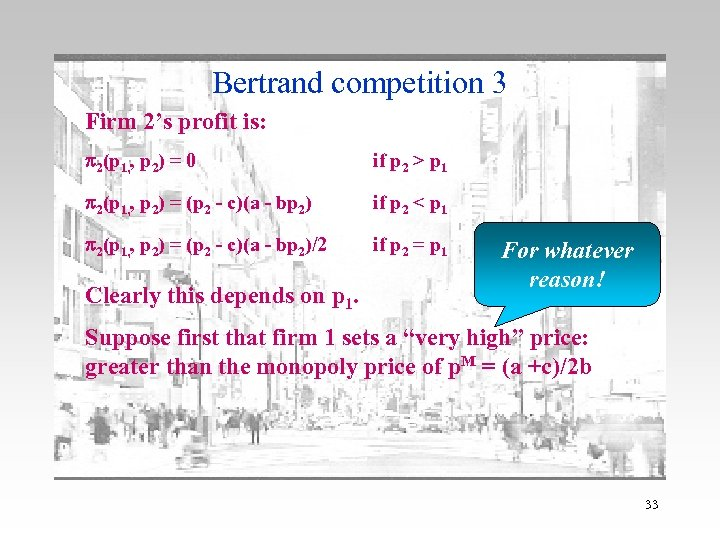Bertrand competition 3 Firm 2’s profit is: p 2(p 1, , p 2) = 0 if p 2 > p 1 p 2(p 1, , p 2) = (p 2 - c)(a - bp 2) if p 2 < p 1 p 2(p 1, , p 2) = (p 2 - c)(a - bp 2)/2 if p 2 = p 1 Clearly this depends on p 1. For whatever reason! Suppose first that firm 1 sets a “very high” price: greater than the monopoly price of p. M = (a +c)/2 b 33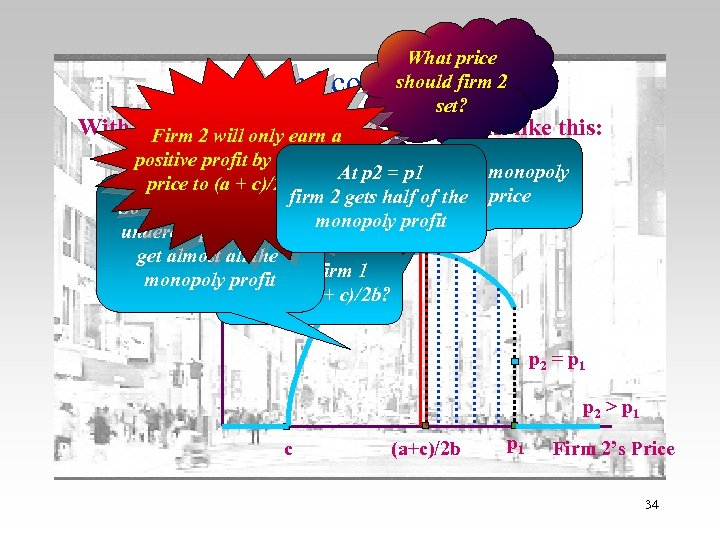What price should firm 2 set? Bertrand competition 4 With p 1 > (a 2+ c)/2 b, earn a 2’s profit looks like this: Firm will only Firm positive profit by cutting its Firm 2’s Profit At p 2 = p 1 The monopoly price to (a + c)/2 b or less firm 2 gets half of the price So firm 2 should just monopoly profit undercut p 1 a bit and p 2 < p 1 get almost all the What monopoly profit if firm 1 prices at (a + c)/2 b? p 2 = p 1 p 2 > p 1 c (a+c)/2 b p 1 Firm 2’s Price 34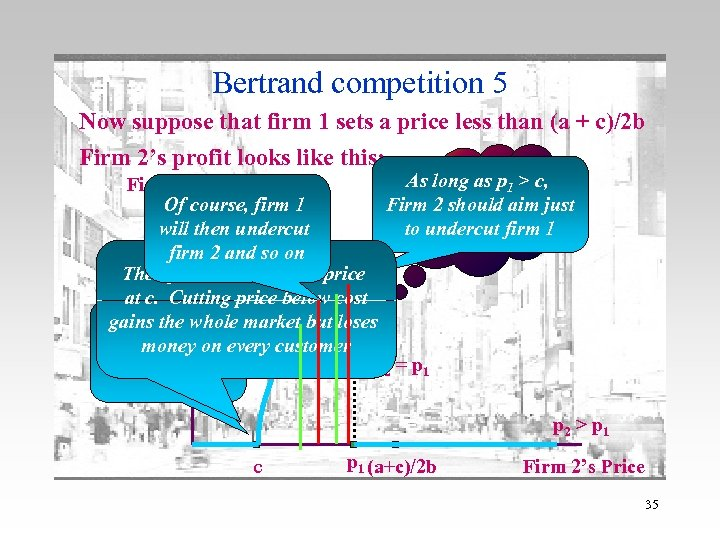Bertrand competition 5 Now suppose that firm 1 sets a price less than (a + c)/2 b Firm 2’s profit looks like this: What price As long as p 1 > c, Firm 2’s Profit Of course, firm 1 Firm 2 should aim just should firm 2 to undercut firm 1 will then undercut set now? firm 2 and so on p 2 < p 1 Then firm 2 should also price at c. Cutting price below cost gains the whole market but loses What if firm 1 every customer money on prices at c? p 2 = p 1 p 2 > p 1 c p 1 (a+c)/2 b Firm 2’s Price 35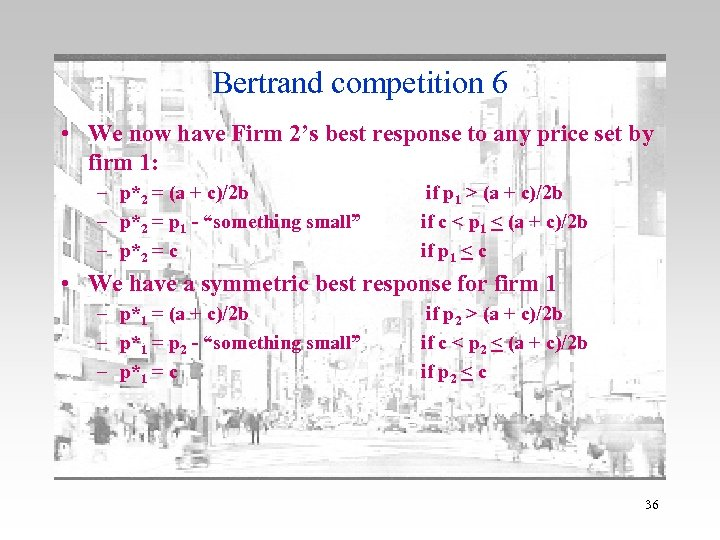Bertrand competition 6 • We now have Firm 2’s best response to any price set by firm 1: – p*2 = (a + c)/2 b – p*2 = p 1 - “something small” – p*2 = c if p 1 > (a + c)/2 b if c < p 1 < (a + c)/2 b if p 1 < c • We have a symmetric best response for firm 1 – p*1 = (a + c)/2 b – p*1 = p 2 - “something small” – p*1 = c if p 2 > (a + c)/2 b if c < p 2 < (a + c)/2 b if p 2 < c 36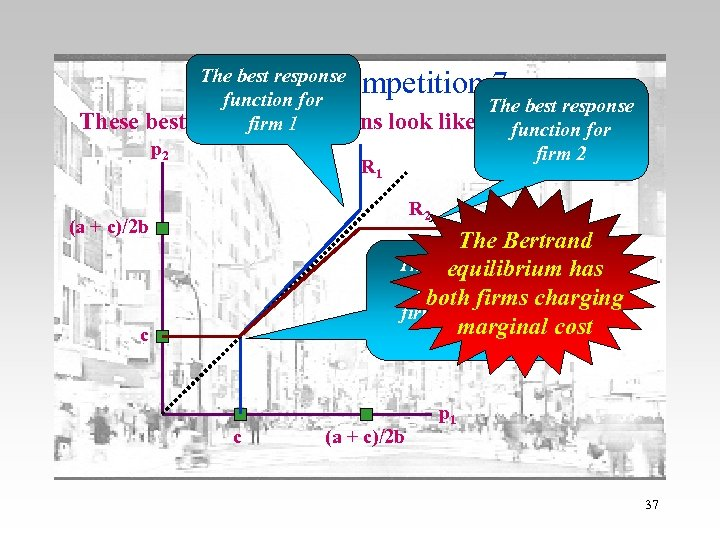Bertrand competition 7 These best The best response function for response functions firm 1 p 2 look like R 1 The best response this function for firm 2 R 2 (a + c)/2 b The Bertrand The equilibrium has is with both charging both firms pricing at marginal cost c c c (a + c)/2 b p 1 37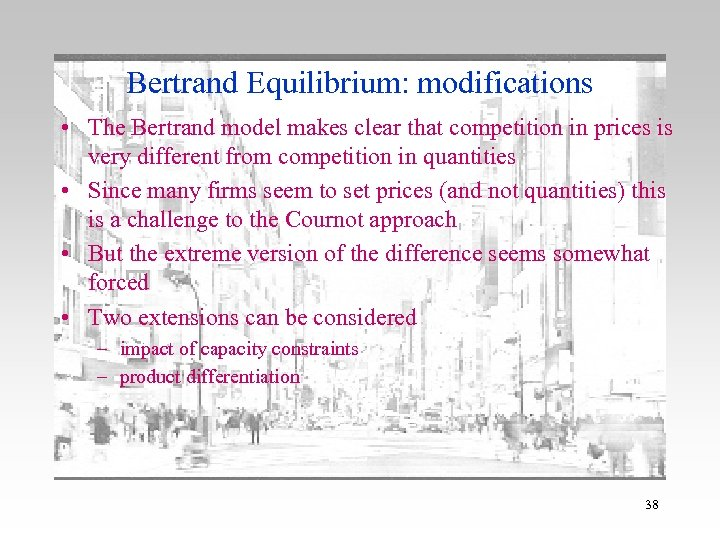Bertrand Equilibrium: modifications • The Bertrand model makes clear that competition in prices is very different from competition in quantities • Since many firms seem to set prices (and not quantities) this is a challenge to the Cournot approach • But the extreme version of the difference seems somewhat forced • Two extensions can be considered – impact of capacity constraints – product differentiation 38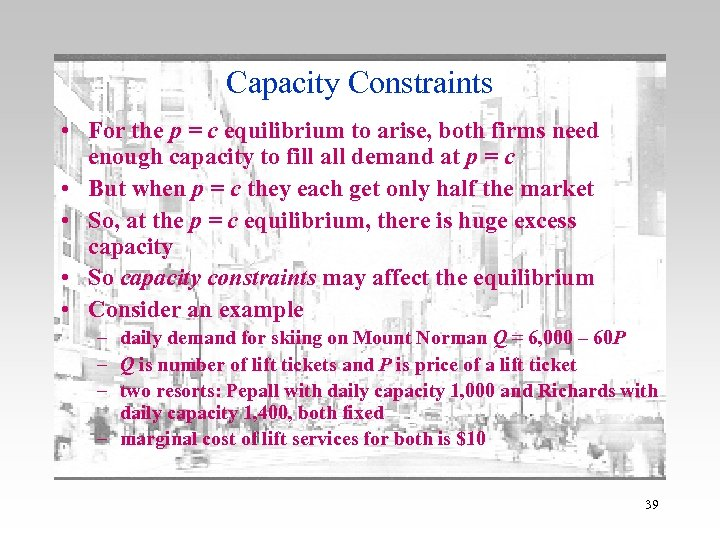Capacity Constraints • For the p = c equilibrium to arise, both firms need enough capacity to fill all demand at p = c • But when p = c they each get only half the market • So, at the p = c equilibrium, there is huge excess capacity • So capacity constraints may affect the equilibrium • Consider an example – daily demand for skiing on Mount Norman Q = 6, 000 – 60 P – Q is number of lift tickets and P is price of a lift ticket – two resorts: Pepall with daily capacity 1, 000 and Richards with daily capacity 1, 400, both fixed – marginal cost of lift services for both is \$10 39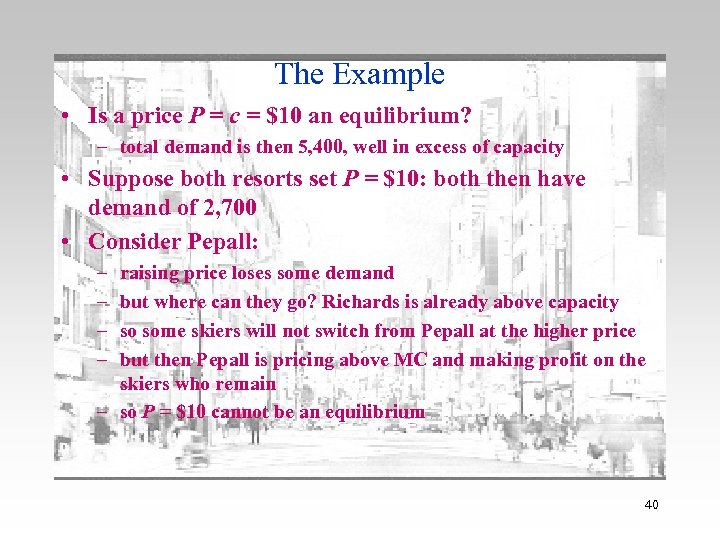The Example • Is a price P = c = \$10 an equilibrium? – total demand is then 5, 400, well in excess of capacity • Suppose both resorts set P = \$10: both then have demand of 2, 700 • Consider Pepall: – – raising price loses some demand but where can they go? Richards is already above capacity so some skiers will not switch from Pepall at the higher price but then Pepall is pricing above MC and making profit on the skiers who remain – so P = \$10 cannot be an equilibrium 40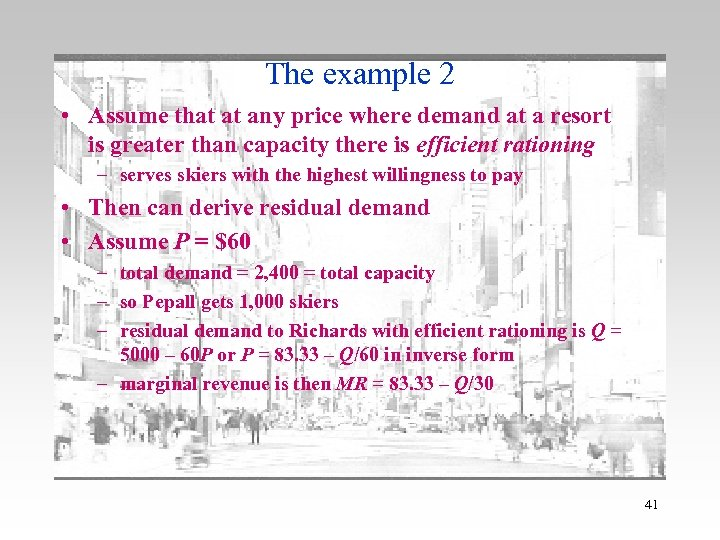The example 2 • Assume that at any price where demand at a resort is greater than capacity there is efficient rationing – serves skiers with the highest willingness to pay • Then can derive residual demand • Assume P = \$60 – total demand = 2, 400 = total capacity – so Pepall gets 1, 000 skiers – residual demand to Richards with efficient rationing is Q = 5000 – 60 P or P = 83. 33 – Q/60 in inverse form – marginal revenue is then MR = 83. 33 – Q/30 41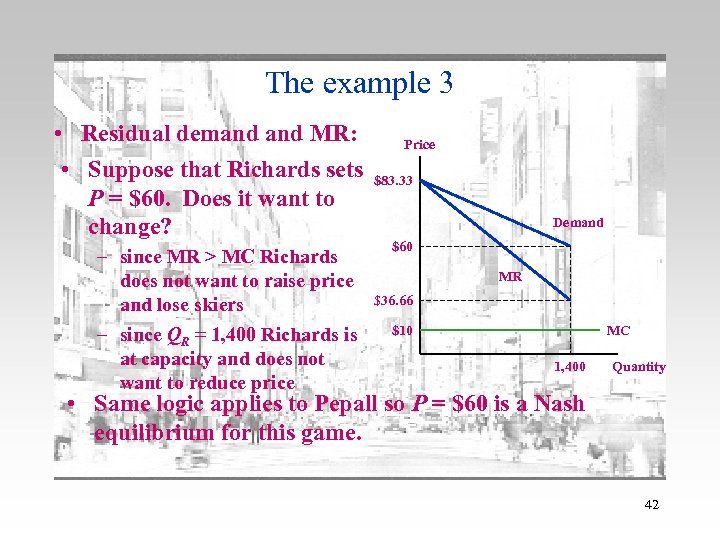The example 3 • Residual demand MR: • Suppose that Richards sets P = \$60. Does it want to change? – since MR > MC Richards does not want to raise price and lose skiers – since QR = 1, 400 Richards is at capacity and does not want to reduce price Price \$83. 33 Demand \$60 MR \$36. 66 \$10 MC 1, 400 Quantity • Same logic applies to Pepall so P = \$60 is a Nash equilibrium for this game. 42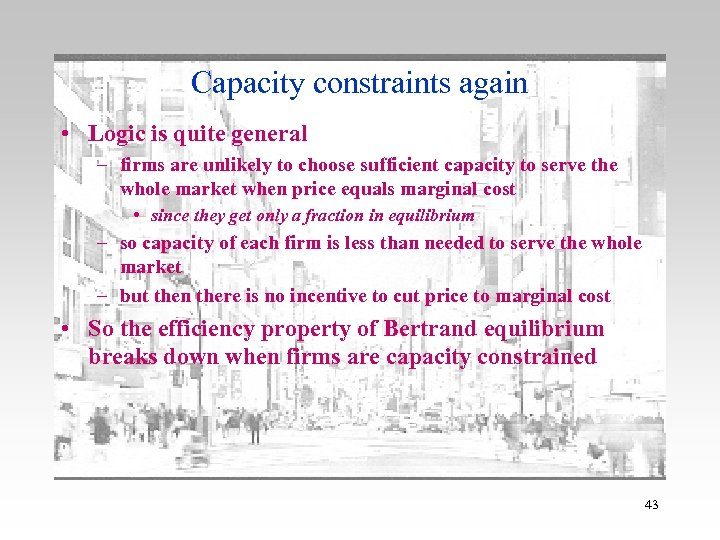Capacity constraints again • Logic is quite general – firms are unlikely to choose sufficient capacity to serve the whole market when price equals marginal cost • since they get only a fraction in equilibrium – so capacity of each firm is less than needed to serve the whole market – but then there is no incentive to cut price to marginal cost • So the efficiency property of Bertrand equilibrium breaks down when firms are capacity constrained 43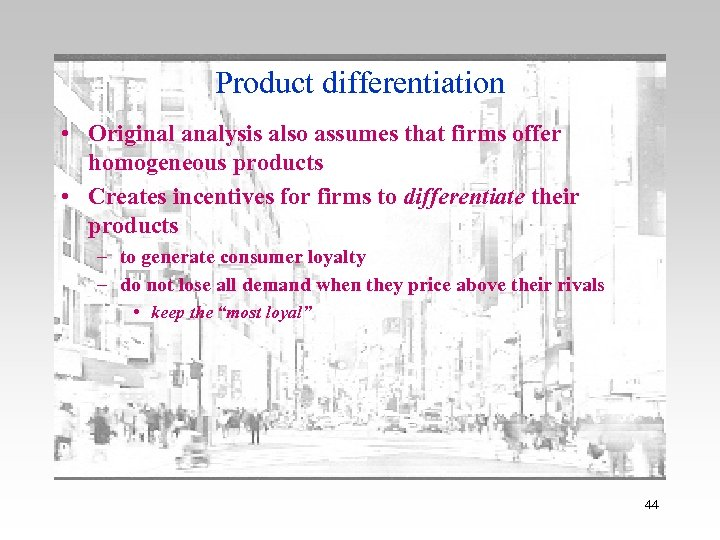Product differentiation • Original analysis also assumes that firms offer homogeneous products • Creates incentives for firms to differentiate their products – to generate consumer loyalty – do not lose all demand when they price above their rivals • keep the “most loyal” 44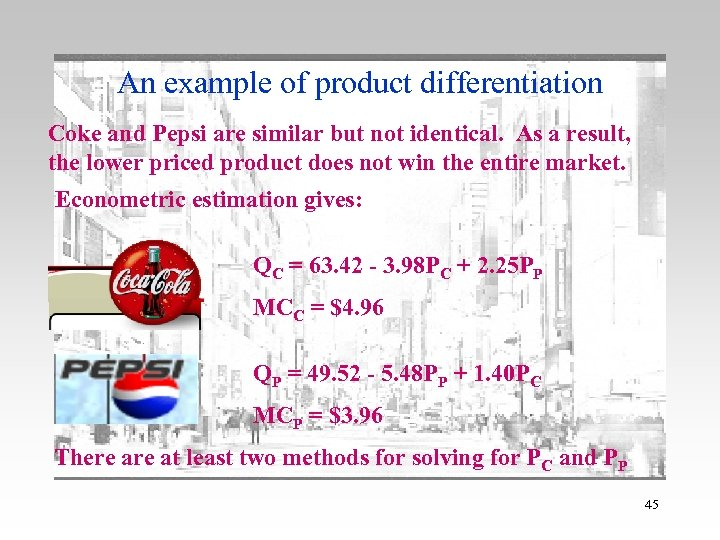An example of product differentiation Coke and Pepsi are similar but not identical. As a result, the lower priced product does not win the entire market. Econometric estimation gives: QC = 63. 42 - 3. 98 PC + 2. 25 PP MCC = \$4. 96 QP = 49. 52 - 5. 48 PP + 1. 40 PC MCP = \$3. 96 There at least two methods for solving for PC and PP 45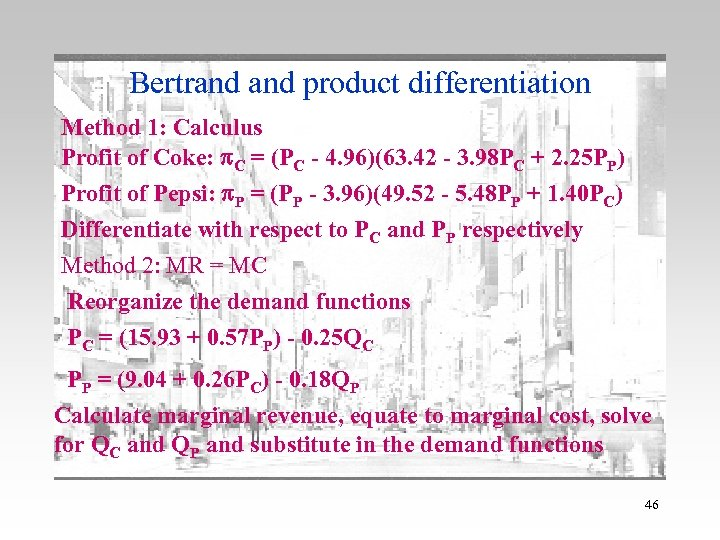Bertrand product differentiation Method 1: Calculus Profit of Coke: p. C = (PC - 4. 96)(63. 42 - 3. 98 PC + 2. 25 PP) Profit of Pepsi: p. P = (PP - 3. 96)(49. 52 - 5. 48 PP + 1. 40 PC) Differentiate with respect to PC and PP respectively Method 2: MR = MC Reorganize the demand functions PC = (15. 93 + 0. 57 PP) - 0. 25 QC PP = (9. 04 + 0. 26 PC) - 0. 18 QP Calculate marginal revenue, equate to marginal cost, solve for QC and QP and substitute in the demand functions 46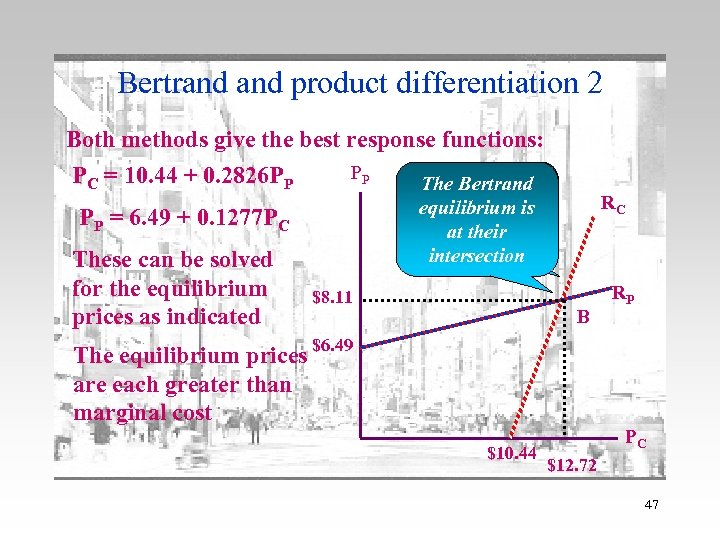Bertrand product differentiation 2 Both methods give the best response functions: PC = 10. 44 + 0. 2826 PP PP PP = 6. 49 + 0. 1277 PC These can be solved for the equilibrium prices as indicated The Bertrand Note that these equilibrium is are upward at their sloping intersection \$8. 11 RC B The equilibrium prices \$6. 49 are each greater than marginal cost \$10. 44 RP PC \$12. 72 47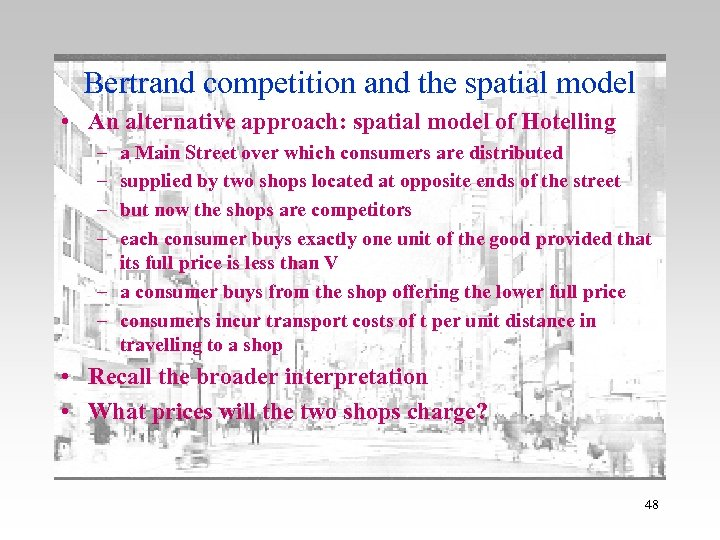Bertrand competition and the spatial model • An alternative approach: spatial model of Hotelling – – a Main Street over which consumers are distributed supplied by two shops located at opposite ends of the street but now the shops are competitors each consumer buys exactly one unit of the good provided that its full price is less than V – a consumer buys from the shop offering the lower full price – consumers incur transport costs of t per unit distance in travelling to a shop • Recall the broader interpretation • What prices will the two shops charge? 48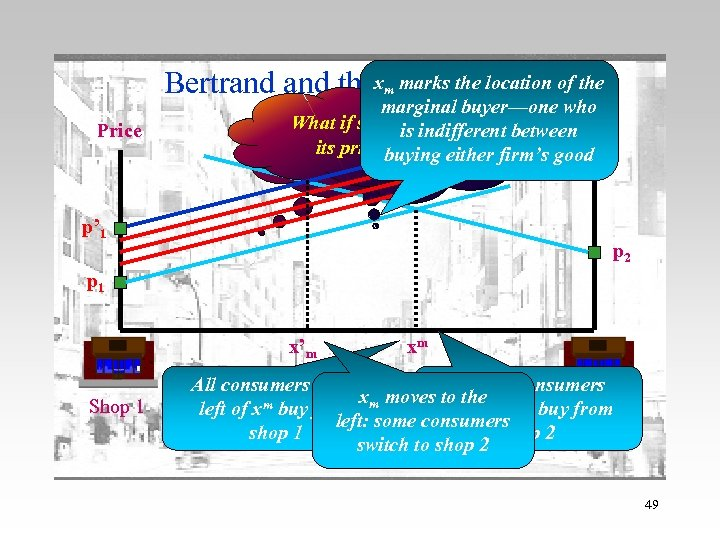Bertrand thexm marks themodel of the spatial location Price marginal buyer—one who Assume that indifferent What if shop is raises 1 sets between 1 shop Price p 1 and shop 2 either firm’s good its price? buying sets price p 2 p’ 1 p 2 p 1 x’m Shop 1 xm All consumers to the And all consumers xm moves to the left of xm buy from to the right buy. Shop 2 from left: some consumers shop 1 shop 2 switch to shop 2 49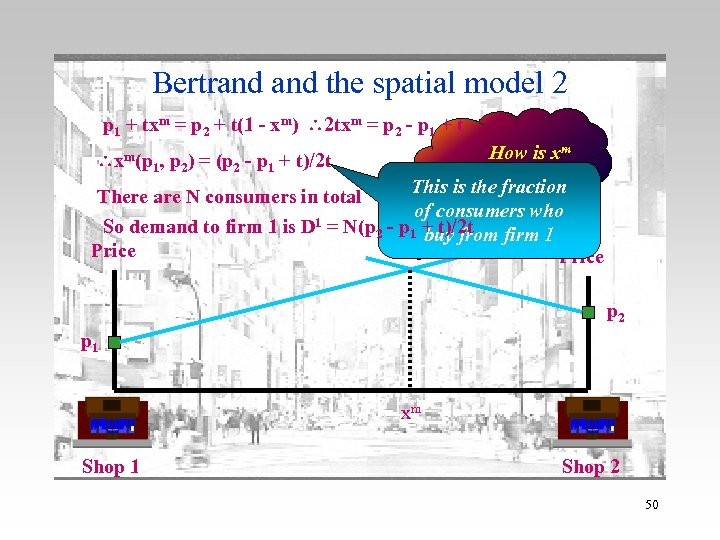Bertrand the spatial model 2 p 1 + txm = p 2 + t(1 - xm) 2 txm = p 2 - p 1 + t xm(p 1, p 2) = (p 2 - p 1 + t)/2 t How is xm determined? This is the fraction There are N consumers in total of consumers who 1 = N(p - p + t)/2 t So demand to firm 1 is D 2 1 buy from firm 1 Price p 2 p 1 xm Shop 1 Shop 2 50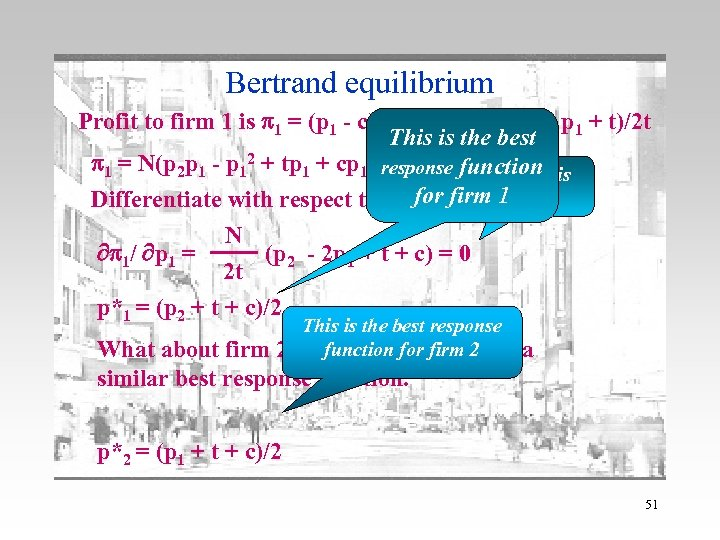Bertrand equilibrium Profit to firm 1 is p 1 = (p 1 - c)D 1 = N(p 1 - c)(p 2 - p 1 + t)/2 t This is the best p 1 = N(p 2 p 1 - p 12 + tp 1 + cp 1 -response functionthis cp 2 -ct)/2 t Solve Differentiate with respect to p 1 for firm 1 for p 1 N p 1/ p 1 = (p 2 - 2 p 1 + t + c) = 0 2 t p*1 = (p 2 + t + c)/2 This is the best response function for firm 2? By symmetry, it has What about similar best response function. a p*2 = (p 1 + t + c)/2 51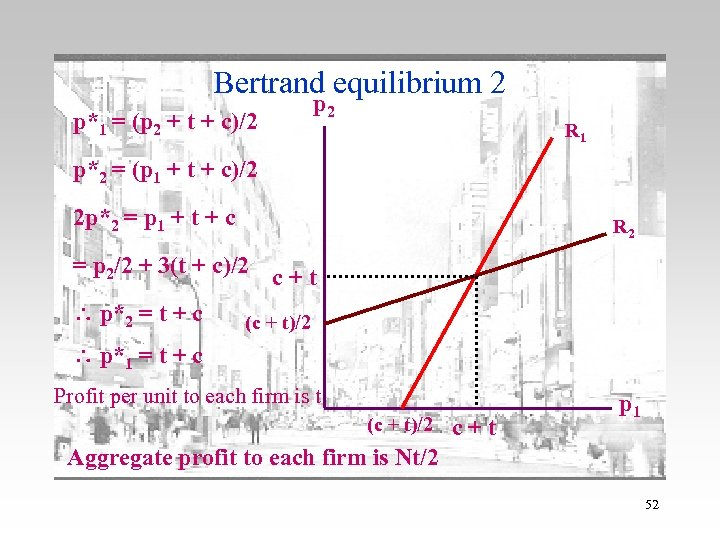Bertrand equilibrium 2 p*1 = (p 2 + t + c)/2 p 2 R 1 p*2 = (p 1 + t + c)/2 2 p*2 = p 1 + t + c R 2 = p 2/2 + 3(t + c)/2 c + t p*2 = t + c (c + t)/2 p*1 = t + c Profit per unit to each firm is t (c + t)/2 c+t p 1 Aggregate profit to each firm is Nt/2 52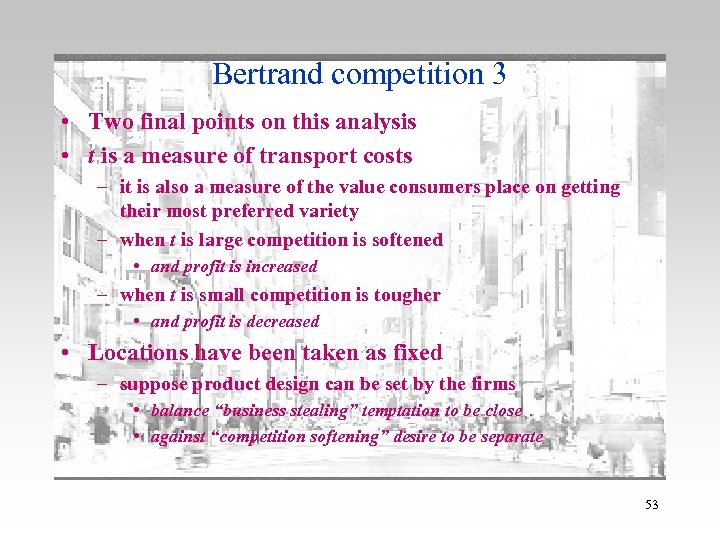Bertrand competition 3 • Two final points on this analysis • t is a measure of transport costs – it is also a measure of the value consumers place on getting their most preferred variety – when t is large competition is softened • and profit is increased – when t is small competition is tougher • and profit is decreased • Locations have been taken as fixed – suppose product design can be set by the firms • balance “business stealing” temptation to be close • against “competition softening” desire to be separate 53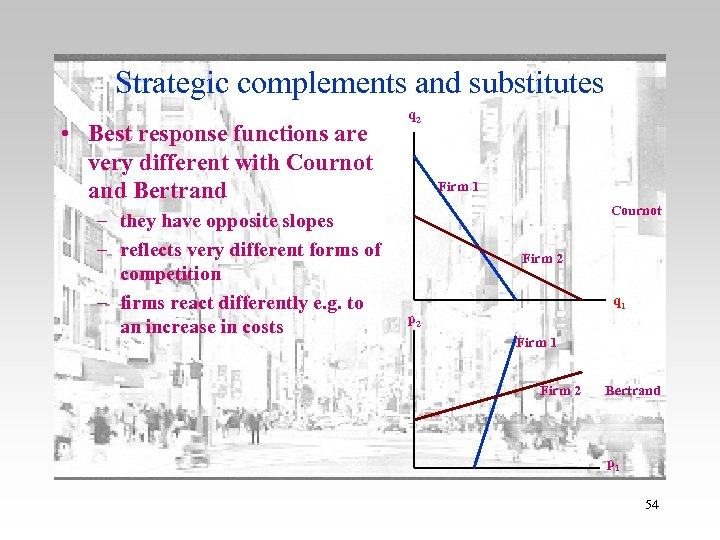Strategic complements and substitutes • Best response functions are very different with Cournot and Bertrand – they have opposite slopes – reflects very different forms of competition – firms react differently e. g. to an increase in costs q 2 Firm 1 Cournot Firm 2 q 1 p 2 Firm 1 Firm 2 Bertrand p 1 54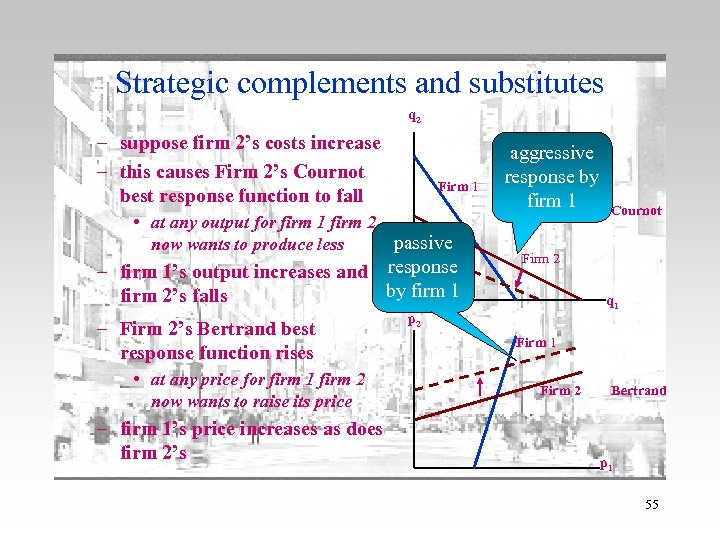Strategic complements and substitutes q 2 – suppose firm 2’s costs increase – this causes Firm 2’s Cournot best response function to fall Firm 1 • at any output for firm 1 firm 2 passive now wants to produce less – firm 1’s output increases and response by firm 1 firm 2’s falls – Firm 2’s Bertrand best response function rises • at any price for firm 1 firm 2 now wants to raise its price – firm 1’s price increases as does firm 2’s aggressive response by firm 1 Cournot Firm 2 q 1 p 2 Firm 1 Firm 2 Bertrand p 1 55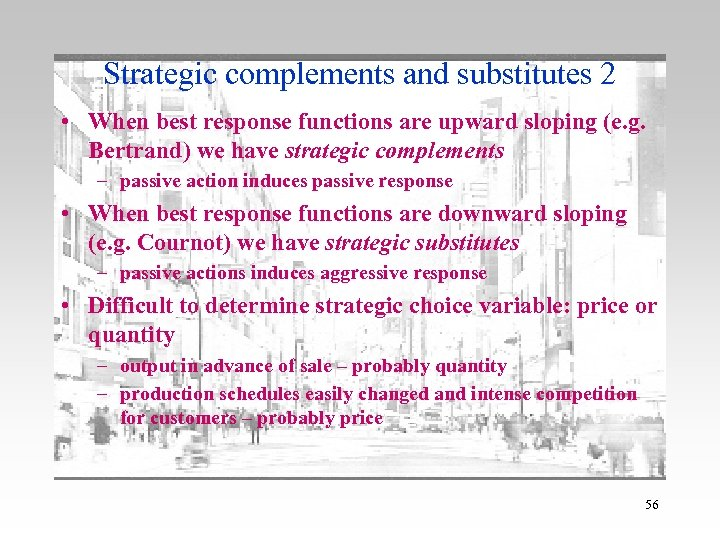Strategic complements and substitutes 2 • When best response functions are upward sloping (e. g. Bertrand) we have strategic complements – passive action induces passive response • When best response functions are downward sloping (e. g. Cournot) we have strategic substitutes – passive actions induces aggressive response • Difficult to determine strategic choice variable: price or quantity – output in advance of sale – probably quantity – production schedules easily changed and intense competition for customers – probably price 56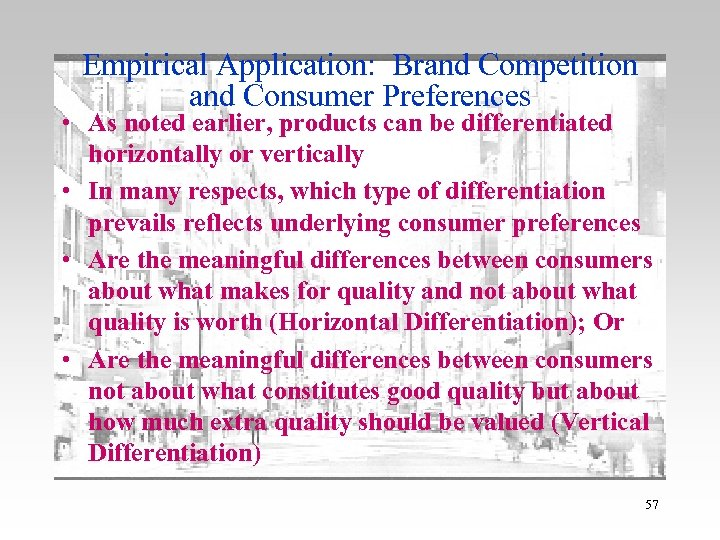Empirical Application: Brand Competition and Consumer Preferences • As noted earlier, products can be differentiated horizontally or vertically • In many respects, which type of differentiation prevails reflects underlying consumer preferences • Are the meaningful differences between consumers about what makes for quality and not about what quality is worth (Horizontal Differentiation); Or • Are the meaningful differences between consumers not about what constitutes good quality but about how much extra quality should be valued (Vertical Differentiation) 57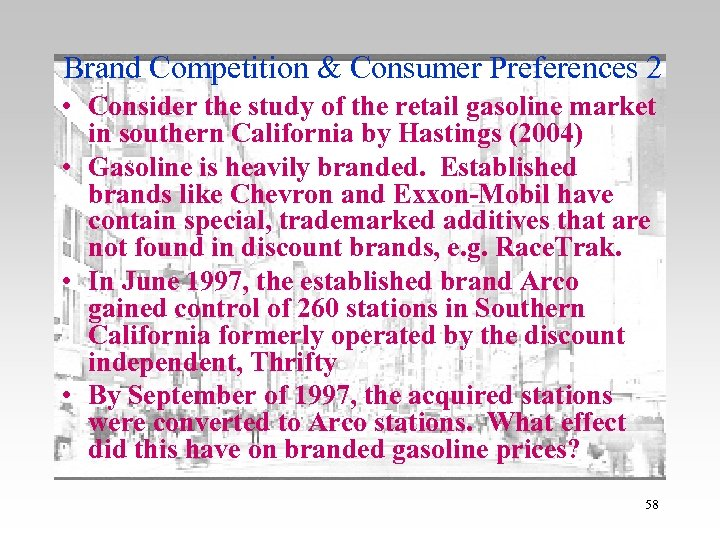Brand Competition & Consumer Preferences 2 • Consider the study of the retail gasoline market in southern California by Hastings (2004) • Gasoline is heavily branded. Established brands like Chevron and Exxon-Mobil have contain special, trademarked additives that are not found in discount brands, e. g. Race. Trak. • In June 1997, the established brand Arco gained control of 260 stations in Southern California formerly operated by the discount independent, Thrifty • By September of 1997, the acquired stations were converted to Arco stations. What effect did this have on branded gasoline prices? 58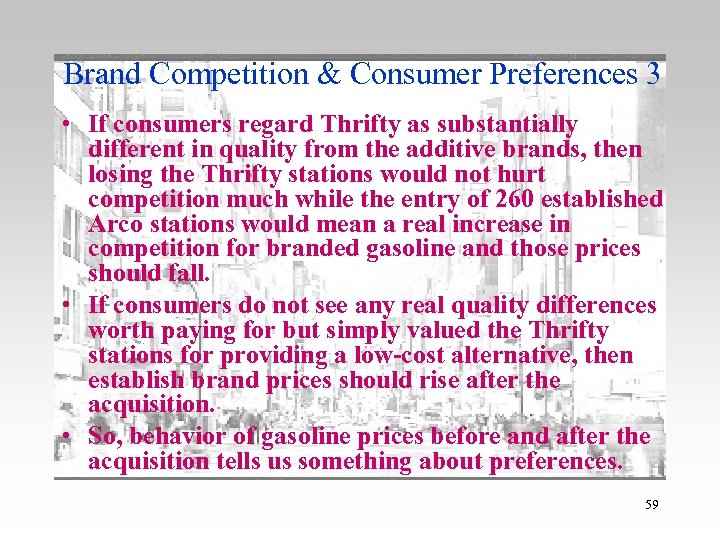Brand Competition & Consumer Preferences 3 • If consumers regard Thrifty as substantially different in quality from the additive brands, then losing the Thrifty stations would not hurt competition much while the entry of 260 established Arco stations would mean a real increase in competition for branded gasoline and those prices should fall. • If consumers do not see any real quality differences worth paying for but simply valued the Thrifty stations for providing a low-cost alternative, then establish brand prices should rise after the acquisition. • So, behavior of gasoline prices before and after the acquisition tells us something about preferences. 59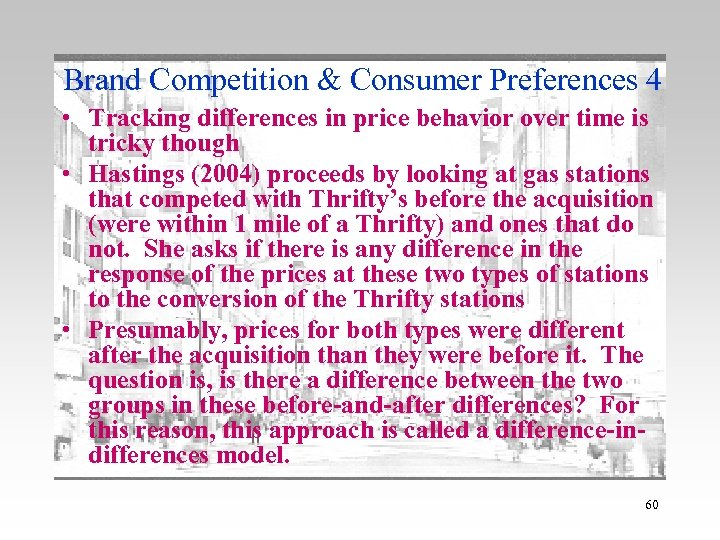Brand Competition & Consumer Preferences 4 • Tracking differences in price behavior over time is tricky though • Hastings (2004) proceeds by looking at gas stations that competed with Thrifty’s before the acquisition (were within 1 mile of a Thrifty) and ones that do not. She asks if there is any difference in the response of the prices at these two types of stations to the conversion of the Thrifty stations • Presumably, prices for both types were different after the acquisition than they were before it. The question is, is there a difference between the two groups in these before-and-after differences? For this reason, this approach is called a difference-indifferences model. 60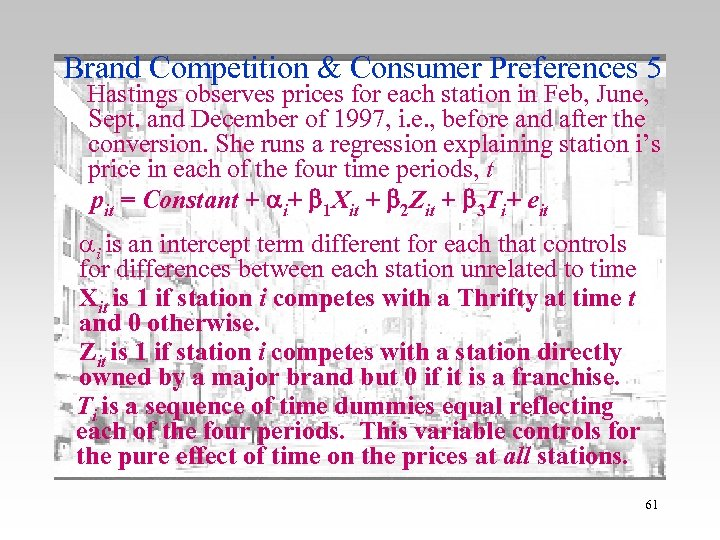Brand Competition & Consumer Preferences 5 Hastings observes prices for each station in Feb, June, Sept. and December of 1997, i. e. , before and after the conversion. She runs a regression explaining station i’s price in each of the four time periods, t pit = Constant + i+ 1 Xit + 2 Zit + 3 Ti+ eit i is an intercept term different for each that controls for differences between each station unrelated to time Xit is 1 if station i competes with a Thrifty at time t and 0 otherwise. Zit is 1 if station i competes with a station directly owned by a major brand but 0 if it is a franchise. Ti is a sequence of time dummies equal reflecting each of the four periods. This variable controls for the pure effect of time on the prices at all stations. 61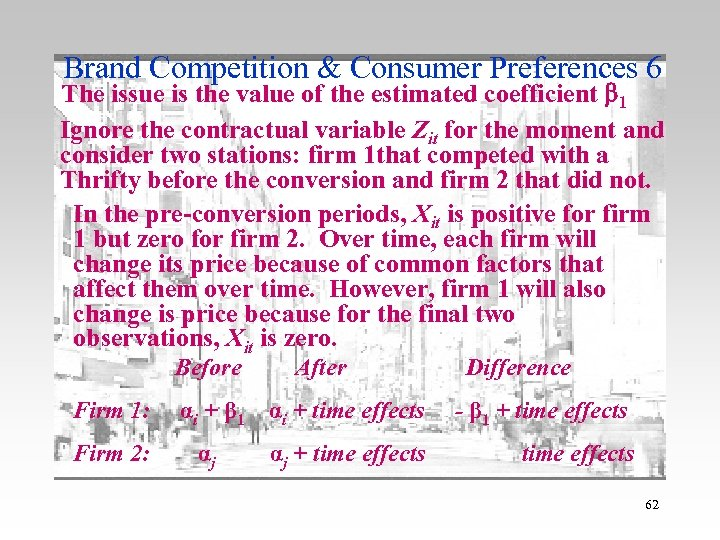Brand Competition & Consumer Preferences 6 The issue is the value of the estimated coefficient 1 Ignore the contractual variable Zit for the moment and consider two stations: firm 1 that competed with a Thrifty before the conversion and firm 2 that did not. In the pre-conversion periods, Xit is positive for firm 1 but zero for firm 2. Over time, each firm will change its price because of common factors that affect them over time. However, firm 1 will also change is price because for the final two observations, Xit is zero. Before Firm 1: Firm 2: After αi + β 1 αi + time effects αj αj + time effects Difference - β 1 + time effects 62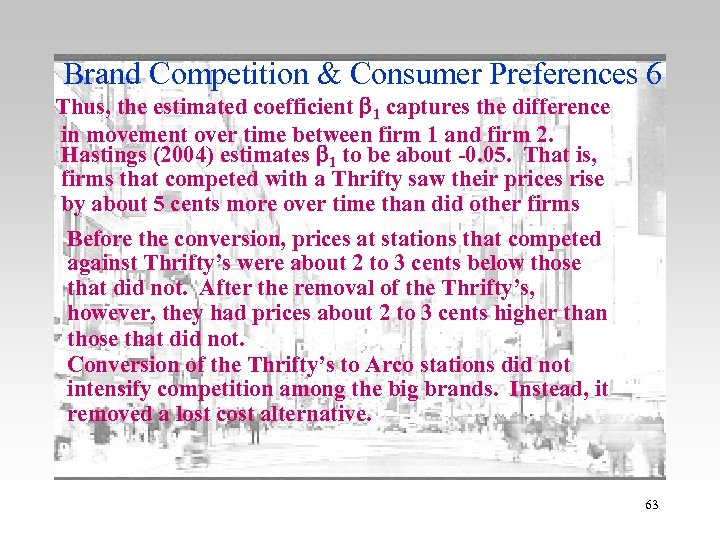Brand Competition & Consumer Preferences 6 Thus, the estimated coefficient 1 captures the difference in movement over time between firm 1 and firm 2. Hastings (2004) estimates 1 to be about -0. 05. That is, firms that competed with a Thrifty saw their prices rise by about 5 cents more over time than did other firms Before the conversion, prices at stations that competed against Thrifty’s were about 2 to 3 cents below those that did not. After the removal of the Thrifty’s, however, they had prices about 2 to 3 cents higher than those that did not. Conversion of the Thrifty’s to Arco stations did not intensify competition among the big brands. Instead, it removed a lost cost alternative. 63